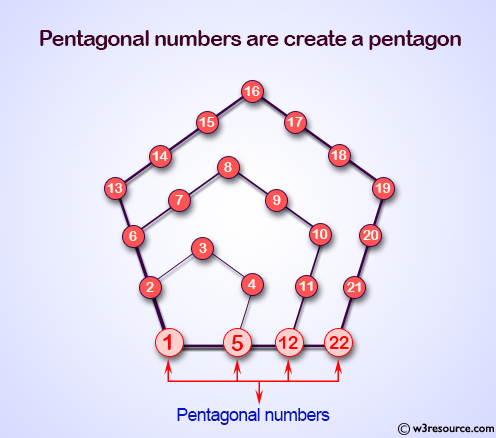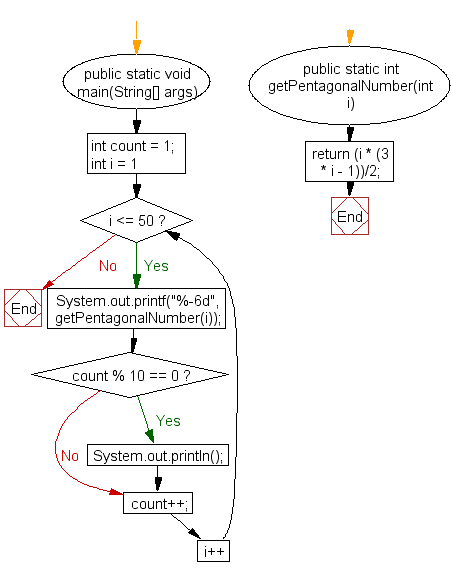﻿ Java exercises: Display the first 50 pentagonal numbers - w3resource# Java Method Exercises: Display the first 50 pentagonal numbers

## Java Method: Exercise-7 with Solution

Write a Java method to display the first 50 pentagonal numbers.

A pentagonal number is a figurate number that extends the concept of triangular and square numbers to the pentagon, but, unlike the first two, the patterns involved in the construction of pentagonal numbers are not rotationally symmetrical.

Pictorial Presentation:Sample Solution:

Java Code:

``````import java.util.Scanner;
public class Exercise7 {
public static void main(String[] args) {
int count = 1;
for(int i = 1; i <= 50; i++){
System.out.printf("%-6d",getPentagonalNumber(i));
if(count % 10 == 0) System.out.println();
count++;
}
}
public static int getPentagonalNumber(int i) {
return (i * (3 * i - 1))/2;
}
}
```
```

Sample Output:

```1     5     12    22    35    51    70    92    117   145
176   210   247   287   330   376   425   477   532   590
651   715   782   852   925   1001  1080  1162  1247  1335
1426  1520  1617  1717  1820  1926  2035  2147  2262  2380
2501  2625  2752  2882  3015  3151  3290  3432  3577  3725
```

Flowchart:Java Code Editor: